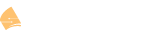# Calculating the amount of networks in a class (A, B or C)

I realize this is somewhat outdated, but i have come across something that is bothering me.

to calculate the amount of networks ( lets use the Class B space for example) we take the network bits (N) and calculate 2^n. except, that isn’t exactly right, because the correct answer is actually 2^14

I understand that this is because the first 2 bits actually don’t change… does anyone have a better equation or just a better way of thinking about/remembering this.

Again i know i am getting hung up on a detail here and it probably boils down to a “training scar”. I am way beyond subnetting, but i am just trying to find the best way to resolve this in my mind.

AAnderson

Hello Austin

When looking at classful networks, we must look at the number of bits in the network portion of the address, but we must also take into account the leading bits. A class A network always has a leading bit of “0”. This means that although there are indeed 8 bits in the network portion, only 7 can be manipulated/modified to denote a network, the leading bit must always be 0. That is why we have 2^7 = 128 networks that range from 0.0.0.0 to 127.0.0.0. Note that 128.0.0.0 is not a class A address because such an address has a leading bit of 1 and not 0.

The same goes for class B networks, where we indeed have 16 bits in the network portion of the address, but class B networks, by definition, have their leading bits set at “10” which means these two bits cannot be used to denote additional networks. That means that we have 16-2=14 bits, so we have 2^14=16384 network addresses, ranging from 128.0.0.0 to 191.255.0.0. Note that the first octet of all numbers ranging from 128 to 191 have the first two bits set to “10” fulfilling the requirement of the definition of the class…

Similarly, class C networks may have 24 bits in the network portion of the address, but the leading bits must be set to “110” which means 2^21 networks can be created.

It’s not always easy to get our heads around the logic behind the classful network scheme, but I hope this clarified it for you somewhat.

I hope this has been helpful!

Laz

1 Like

Thanks,